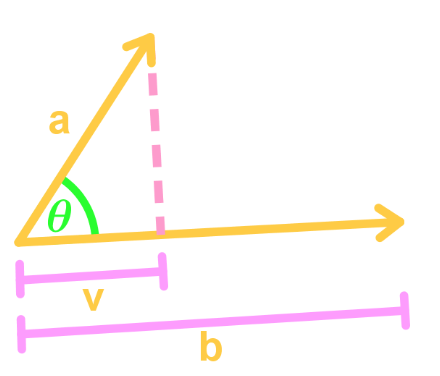# Dot product

### Dot product

#### Lessons

Notes:

Dot Product
Let $u=$ and $v=$. Then the dot product of these two vectors will be:

$u \cdot v = ad + be + df$

Dot Product Property
If the dot product of two vectors $u$ and $v$ gives 0, then the vectors are perpendicular. In other words,

$u \cdot v=0 \to$ perpendicular vectors

Scalar and Vector Projection
Suppose we have two vectors $a$ and $b$. Suppose they create an angle $\theta$ such that we get the following picture:$|v| = \frac{a \cdot b } {|b|}$

To find the vector projection $a$ onto $b$ (which is v), we use the formula:

$v = \frac{a \cdot b}{b \cdot b}b$

Let $u, v, w$ be vectors and $c$ be a scalar. Then the properties of dot products are:
1. $u \cdot u = |u|^2$
2. $u \cdot v = v \cdot u$
3. $u \cdot (v+w) = u \cdot v + u \cdot w$
4. $(cu) \cdot v = u \cdot (cv) = c(u \cdot v)$
• Introduction
Dot Product Overview:
a)
Dot Product and its Special Property
• Multiplying the corresponding entries, and adding
• Dot product = 0 $\to$ vectors are perpendicular

b)
Application to Dot Product
• What is scalar projection? Vector projection
• Formula for scalar projection: $|v| = \frac{a \cdot b}{|b|}$
• Formula for vector projection $v = \frac{a \cdot b}{b \cdot b}b$

c)
More Properties of Dot Product
• Order
• Length
• Distribution
• Scalar

• 1.
Using the Dot Product
Find the dot product of $u = <-1, -2 , 7>$ and $v = <-2,1,-2>$.

• 2.
Find the dot product of $u= <1, -5, -3>$ and $v= <-1, 1, 2>$.

• 3.
Using the Dot Product Property
Suppose two vectors $u = < a, 4, -3>$and $v=<1, 2, 3>$ are perpendicular. Find $a$.

• 4.
Finding Scalar and Vector Projections
Find the scalar and vector projection of $\vec{BA}$ onto $\vec{CA}$ if $A=(1, 0, 2)$, $B=(3, -2, 1)$ and $C=(-4, 1, 5)$.

• 5.
Verifying Properties of Dot Product
Use the two vectors $u=<3, 1, 5>$ and $v=<1,4,-6>$ to show that:

$u \cdot u = |u|^2$

• 6.
Use the 3 vectors $u=<3, 1, 5>$, $v=<1,4,-6>$, and $w=<1, 0, 3>$ to show that:

$u \cdot (v+w)=u \cdot v+ u \cdot w$